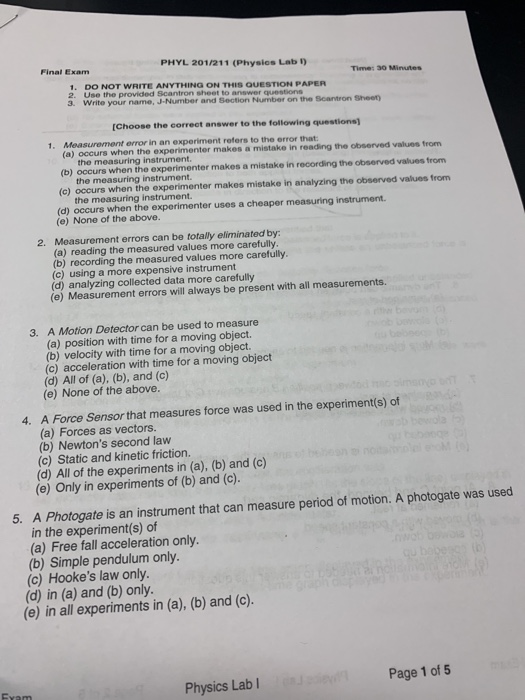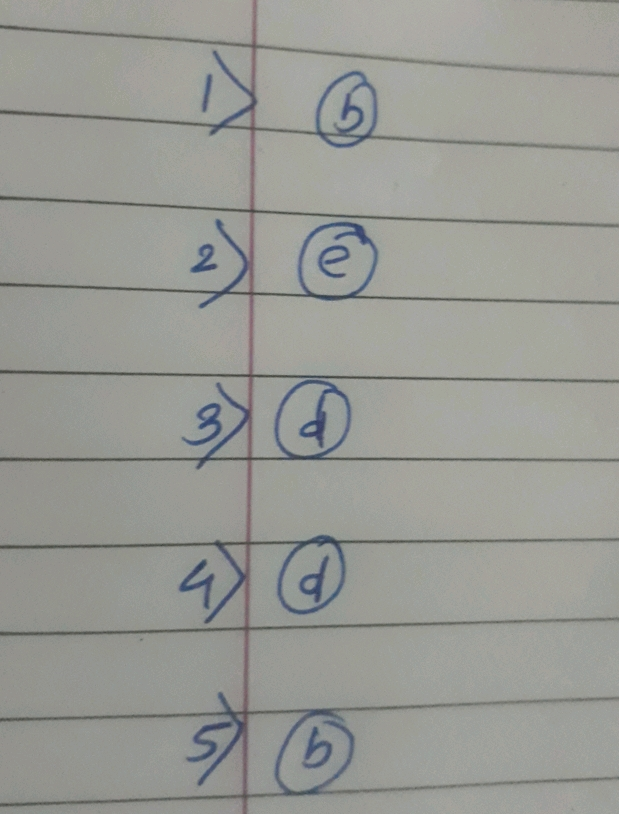Homework Help Question & Answers

# PHYL 201/211 (Physics Lab ) Final Exam Time: 30 Minutes 1. DO NOT WRITE ANYTHING ON THIS QUESTION...PHYL 201/211 (Physics Lab ) Final Exam Time: 30 Minutes 1. DO NOT WRITE ANYTHING ON THIS QUESTION PAPER name, J-Number and Section Number on the Scantron Sheet (Choose the correct answer to the following questions) Measurement error in an experiment refers to the error that (a) occurs when the experimenter makes a mistake in reading the observed values from 1. the measuring instrument. (b) occurs when the experimenter makes a mistake in recording the observed values from the measuring instrument (c) occurs when the experimenter makes mistake in analyzing the observed values from the measuring instrument (d) occurs when the experimenter uses a cheaper measuring instrument. (e) None of the above. 2. Measurement errors can be totally eliminated by: (a) reading the measured values more carefully. (b) recording the measured values more carefully. (c) using a more expensive instrument (d) analyzing collected data more carefully (e) Measurement errors will always be present with all measurements. 3. A Motion Detector can be used to measure (a) position with time for a moving object. (b) velocity with time for a moving object. (c) acceleration with time for a moving object (d) All of (a), (b), and (c) (e) None of the above. 4. A Force Sensor that measures force was used in the experiment(s) of (a) Forces as vectors. (b) Newton's second law (c) Static and kinetic friction. (d) All of the experiments in (a), (b) and (c) (e) Only in experiments of (b) and (c). A Photogate is an instrument that can measure period of motion. A photogate was used in the experiment(s) of (a) Free fall acceleration only. (b) Simple pendulum only. (c) Hooke's law only. (d) in (a) and (b) only. 5. (e) in all experiments in (a), (b) and (c). Physics Lab I Page 1 of 5##### Add Answer of: PHYL 201/211 (Physics Lab ) Final Exam Time: 30 Minutes 1. DO NOT WRITE ANYTHING ON THIS QUESTION...
More Homework Help Questions Additional questions in this topic.

• #### if Steven can mix 20 drinks in 5 minutes, Sue can mix 20 drinks in 10 minutes, and Jack can mix 20 drinks in 15 minutes, how much time will it take all 3 of them working together to mix the 20 drinks

Need Online Homework Help?

Get FREE EXPERT Answers
WITHIN MINUTES
Related Questions